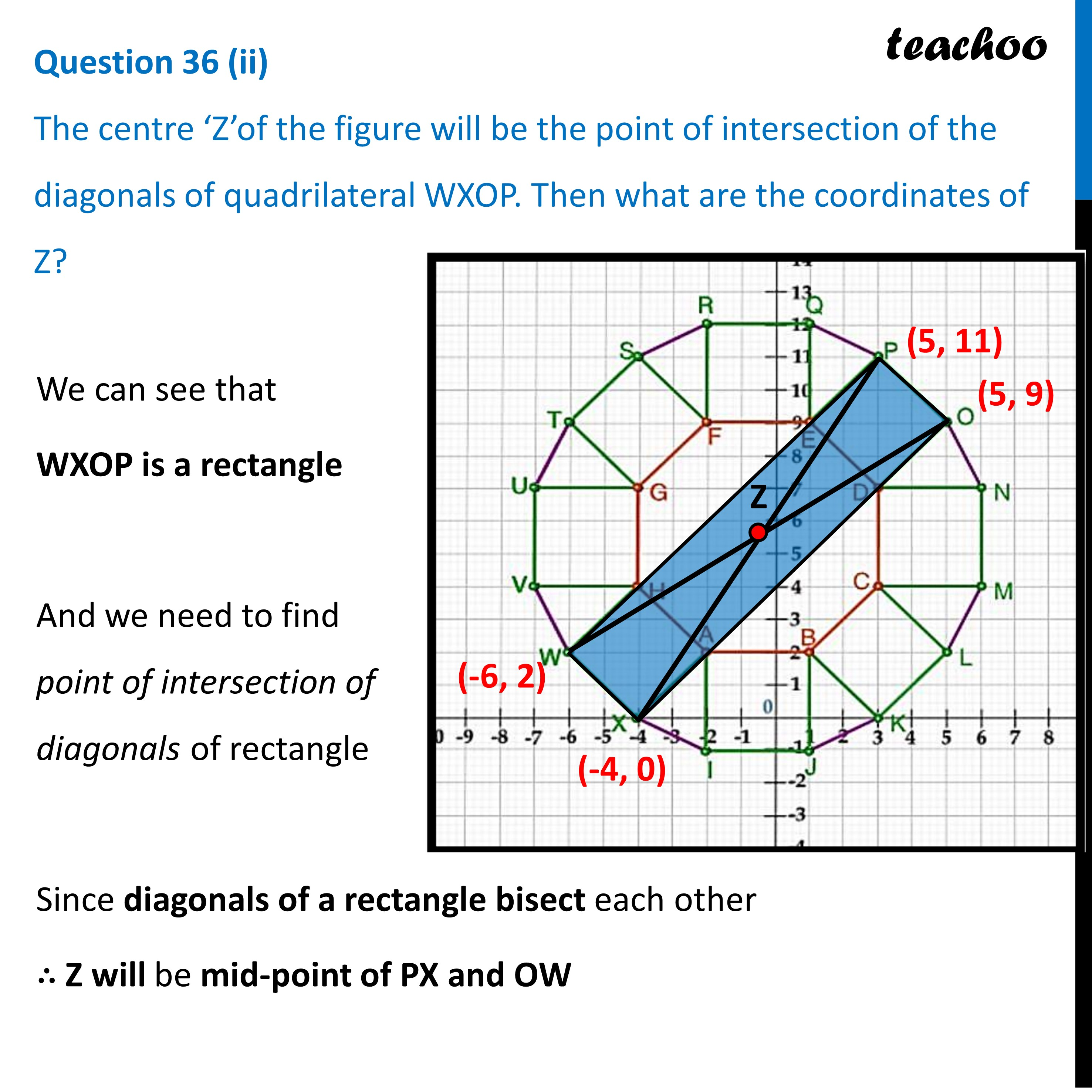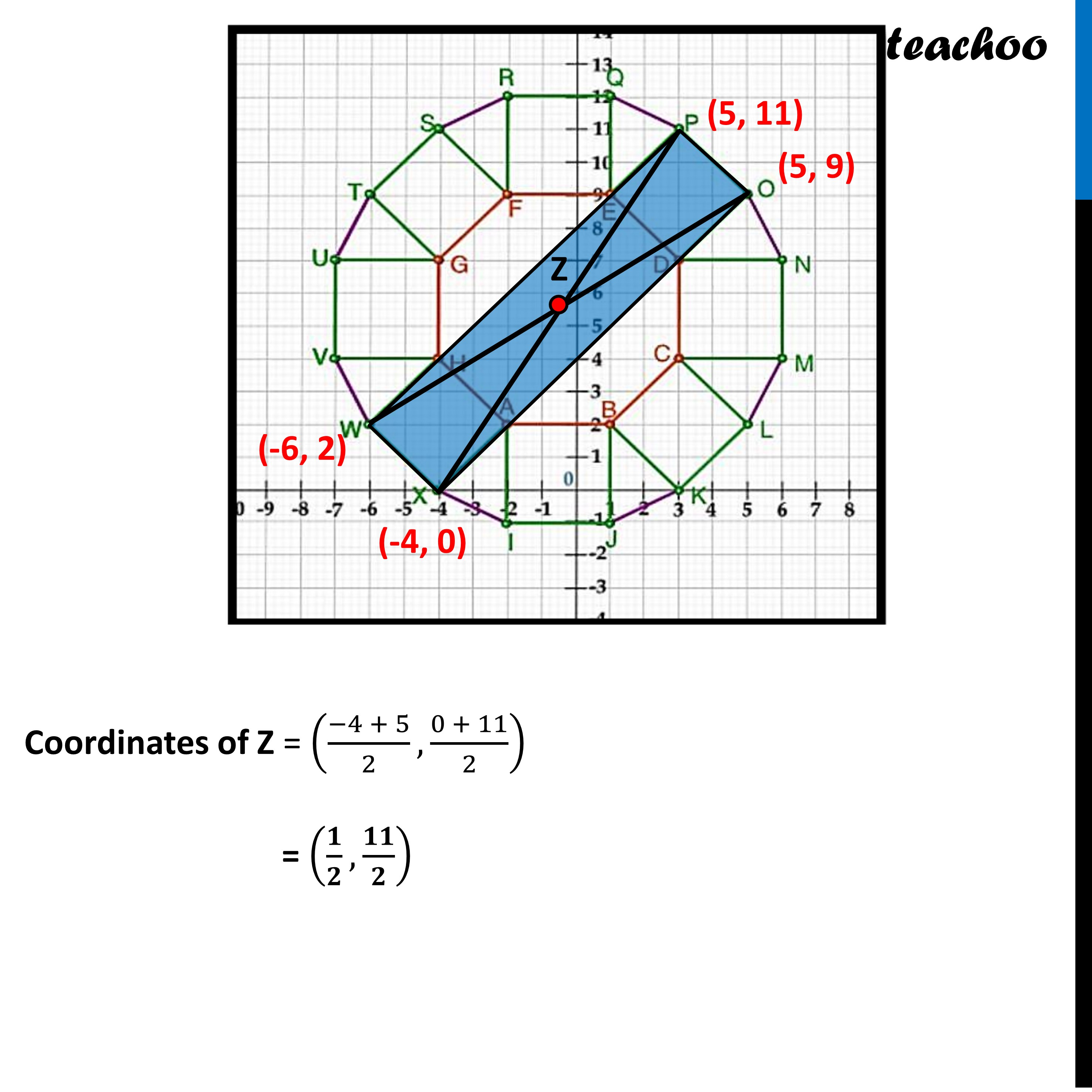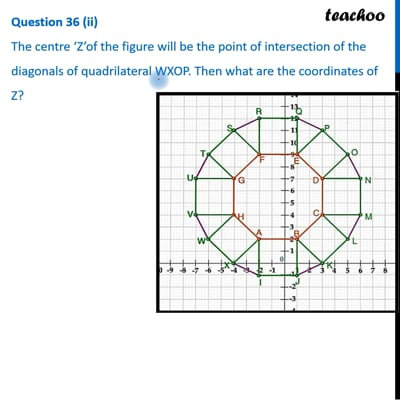CBSE Class 10 Sample Paper for 2023 Boards - Maths Standard

Class 10
Solutions of Sample Papers for Class 10 Boards

## (ii)The centre ‘Z’of the figure will be the point of intersection of the diagonals of quadrilateral WXOP. Then what are the coordinates of Z?This video is only available for Teachoo black users

Learn in your speed, with individual attention - Teachoo Maths 1-on-1 Class

### Transcript

Question 36 (ii) The centre ‘Z’of the figure will be the point of intersection of the diagonals of quadrilateral WXOP. Then what are the coordinates of Z? We can see that WXOP is a rectangle And we need to find point of intersection of diagonals of rectangle Since diagonals of a rectangle bisect each other ∴ Z will be mid-point of PX and OW Coordinates of Z = ((−4 + 5)/2,(0 + 11)/2) = (𝟏/𝟐,𝟏𝟏/𝟐)